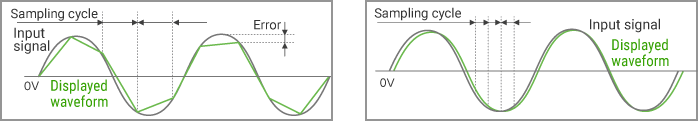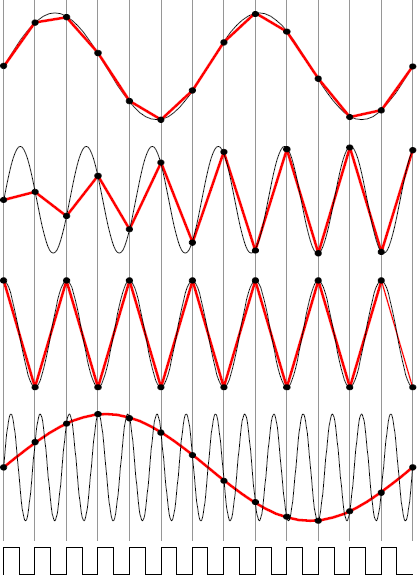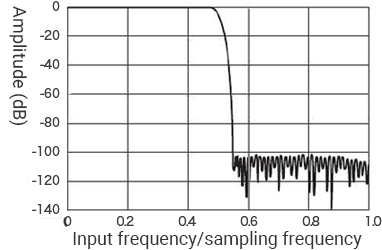# Acceleration Analysis Improvement

The cycle of “sampling,” which is the processing required to convert analog waveforms to digital data, is called the sampling cycle or sampling interval. Electronic measuring instruments measure data at each sampling cycle. Therefore, accurate waveforms may not be measured if the sampling cycle is selected incorrectly. On the other hand, “aliasing,” which is also called “folding noise,” refers to a phenomenon that occurs during the sampling of analog signals. Aliasing has a large impact on FFT analysis and similar operations.
This section explains “sampling” and “filtering,” which are implemented to prevent the effect of aliasing.

## Sampling cycle and aliasing

### Sampling cycle

As shown below, a slow sampling cycle of approximately 5 times the input signal cycle produces a jagged waveform, but a sampling cycle quick enough compared to the input signal cycle (10 times or more) can reproduce a waveform that is almost the same as the input signal.### Aliasing

With the sampling theorem, in order to faithfully regenerate original signals after A/D conversion of AC signals having a frequency of fin, followed by D/A conversion, a sampling frequency fs of at least 2 × fin is required. Caution is required if fs becomes smaller than fin because a non-existing waveform called aliasing is observed in that case.fs > fin
Input signals are faithfully reproduced.

fs ≈ 2 × fin
Input signal frequencies are nearly reproduced, but there are beats in the amplitude.

fs = 2 × fin
In this situation, fin is called the Nyquist frequency.
A waveform with a small amplitude is observed if the sampling phase does not match.

fs < fin
A waveform whose frequency is lower than input frequency fin is observed. This waveform is called the aliasing waveform.

Sampling clock

## Anti-aliasing filter

A spectrum that does not exist may be observed if signals including frequencies over half of the sampling frequency are displayed after sampling and FFT calculation. This spectrum is called folding noise or aliasing.
An anti-aliasing filter is a low-pass filter that dampens frequencies over half of the sampling rate with a high damping characteristic.
Sampling signals that have passed an anti-aliasing filter prevents aliasing from occurring.In this figure, the vertical axis shows the damping rate of input signals while the horizontal axis shows the ratio of the input frequency to the sampling frequency.
You can see that input signals are dampened considerably if the input frequency divided by the sampling frequency is 0.5 or more—that is, the sampling frequency is two times or more the input frequency.

INDEX